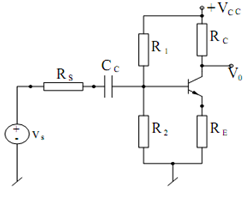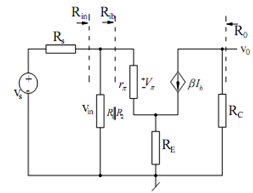## Common-Emitter Amplifier Assignment Help

Assignment Help: >> Transistor Amplifier Circuits - Common-Emitter Amplifier

Common-Emitter Amplifier:

An exemplary common-emitter configuration is illustrated in FigureFigure: An Exemplary Common-emitter Amplifier

For analyzing this circuit, we suppress the DC voltage (by setting Vcc = 0) and replace the transistor by its small signal AC equivalent circuit in terms of rπ and a current source β ib, supposing r0 → ∞, such an equivalent circuit is shown in Figure. The small signal voltage gain Av, of this amplifier may be found as follows :

The loop equation of the base-emitter loop gives :

Vin  = ib  rπ + (ib  + β ib ) RE

so that we may find

Rib = V in  / ib = rπ + (1 + β) RE

The input resistance to the amplifier is, thus, specified by

Ri  = R1 || R2 || Rib

Also,

Vin  = (R i / Ri  + Rs) Vs

and

V0  =- (βib ) RcFigure: AC Small Signal Equivalent Circuit of the Common-emitter Amplifier

Combining the above, we get

A v  = V0   / V in =- β Rc /  [r π + (1 + β) RE]( Ri / Ri  + RS  )

From the above, we see that if Ri >> Rs and (1 + β) RE >> rπ then

AV  ≅    - β Rc/(1 + β) RE  = - RC/ RE  , for large β.

Furthermore, if RE = 0 and RC >> RS

Av  ≅ (β/ r π) RC  ≅ - gm Rc

Thus , It is seen that gain is negative which means that input and output of the amplifier are 180o out of place.#### Assured A++ Grade

Get guaranteed satisfaction & time on delivery in every assignment order you paid with us! We ensure premium quality solution document along with free turntin report!

All rights reserved! Copyrights ©2019-2020 ExpertsMind IT Educational Pvt Ltd